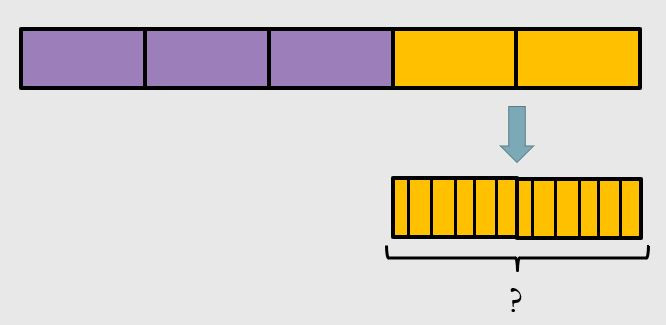Search
•Jessica Kaminski

# Bar Modeling Make Word Problems about Operations

Updated: Jul 30

When students solve word problems, there can be a lot of challenges.

• Maybe students struggle with all the language there is to process in the actual reading of the word problem.

• Maybe students aren't sure what operation to use when solving the problem.

• Maybe students don't know where to start when solving a multi-step problem.

Word problems are just plain challenging. They require students to combine several skills and apply their understanding. One way we can do that is to encourage students to visualize what's happening in the problem.In Grades Kindergarten and 1, students are first exposed to word problems by acting them out. They learn that addition means to combine or put together and subtraction means to take away. Students see that they can act this out with real items and then model it with an operation. This step is KEY when students are developing their understanding.

By the end of Grade 1, students begin to see that there can be differences in how each operation is used. Maybe it's still addition but we can use subtraction to solve. Students in Grade 2 actually explore 15 different addition and subtraction scenarios including comparison problems.By Grades 3 and 4, students are introduced to multiplication and division scenarios again building from conceptual understanding to abstract thinking by first representing equal groups. Students see that division can be represented in two ways: the size of the group or the number of groups. This presents itself in 9 different problem types.Let's recap: Our students have to know 24 different problem types by the end of Grade 4! They begin two-step problems in Grade 2 and apply all four operations in Grade 3! Wow!

This is a HUGE task. It's easy to lean back into old tricks that we think might work- teaching key words and memorization. But let's be real...those are short term fixes. Instead we can look towards a solution that will help our students process what's happening in the problem.Bar models are diagrams that are drawn to help students interpret the math in the problem. They are not new to mathematics and have been called a variety of things such as tape diagrams, tape models, or bar diagrams. These visual models help students solve word problems in a few important ways.

1. Students draw a model that shows a relationship.

2. Students see how that relationship models an operation.

3. Students determine what steps to do by seeing what needs to be solved in their bar model.

4. Students prepare for algebra by placing a question mark for the unknown and solve.

In order to do these, students have to learn HOW to draw them and use them. We can teach them these models with each problem type and help students to feel confident moving forward. Then, students can apply that thinking with multi-step problems, fractions, decimals and so much more!

If you are wanting to implement these with your students, I have two amazing resources just for you. The first is a FREE download for classroom posters showing bar models for each problem types. These will be a lifesaver as you begin learning these alongside your students. You can download them by clicking below:Once you have your FREE download, be sure to check out the 2 Problem Solving Handbooks that will definitely change the way you do word problems. Each book explores the different problem types and then walks you through:

• a concrete example

• a visual example

• a multi-step problem

Oh, and each one comes with video that teaches each word problem to your student! Already HUNDREDS of students are seeing a huge difference in their confidence with word problems!

You can grab your copy below!